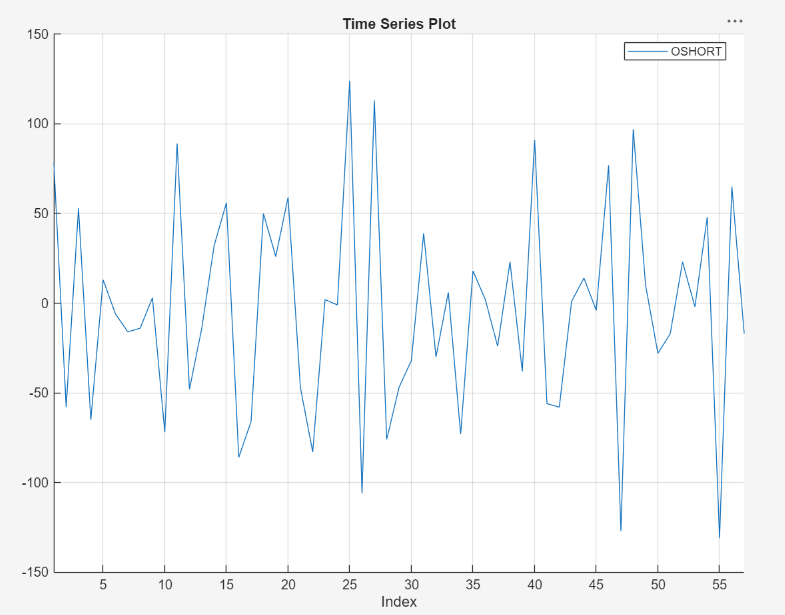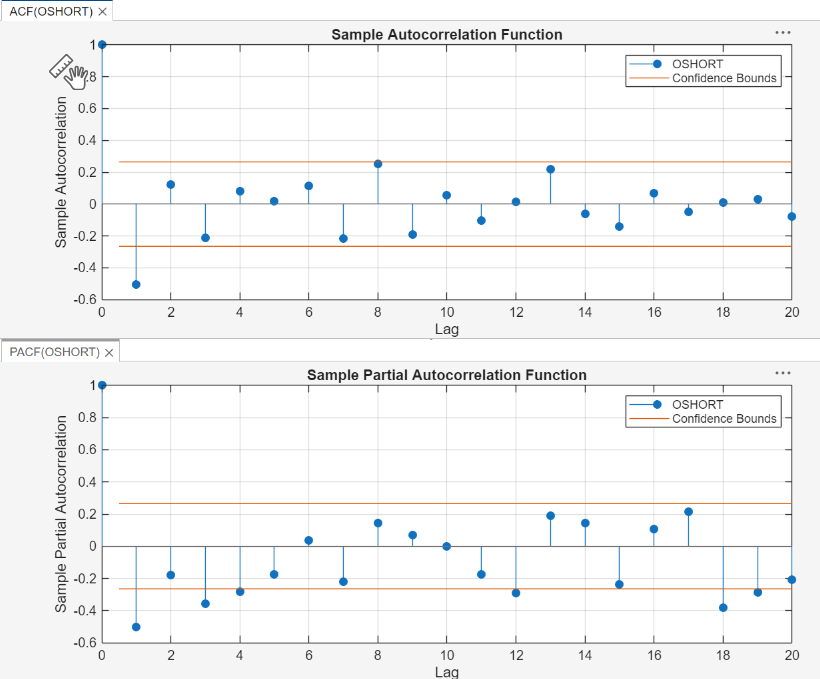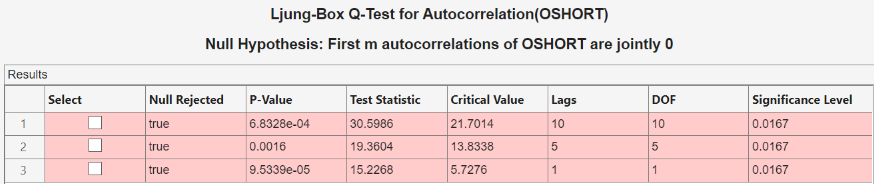## Detect Serial Correlation Using Econometric Modeler App

These examples show how to assess serial correlation by using the Econometric Modeler app. Methods include plotting the autocorrelation function (ACF) and partial autocorrelation function (PACF), and testing for significant lag coefficients using the Ljung-Box Q-test. The data set `Data_Overshort.mat` contains 57 consecutive days of overshorts from a gasoline tank in Colorado.

### Plot ACF and PACF

This example shows how to plot the ACF and PACF of a time series.

At the command line, load the `Data_Overshort.mat` data set.

`load Data_Overshort`

At the command line, open the Econometric Modeler app.

`econometricModeler`

Alternatively, open the app from the apps gallery (see Econometric Modeler).

Import `DataTimeTable` into the app:

1. On the Econometric Modeler tab, in the Import section, click the button.

2. In the Import Data dialog box, in the Import? column, select the check box for the `DataTimeTable` variable.

3. Click .

The variable `OSHORT` appears in the Time Series pane, and its time series plot appears in the Time Series Plot(OSHORT) figure window.The series appears to be stationary.

Close the Time Series Plot(OSHORT) figure window.

Plot the ACF of `OSHORT` by clicking the Plots tab. The ACF appears in the ACF(OSHORT) figure window then clicking ACF.

Plot the PACF of `OSHORT` by clicking the Plots tab then clicking PACF. The PACF appears in the PACF(OSHORT) figure window.

Position the correlograms so that you can view them at the same time by dragging the PACF(OSHORT) figure window to the bottom of the right pane.The sample ACF and PACF exhibit significant autocorrelation (that is, both contain lags that are more than two standard deviations away from 0). The sample ACF shows that the autocorrelation at lag 1 is significant. The sample PACF shows that the autocorrelations at lags 1, 3, and 4 are significant.

The distinct cutoff of the ACF and the more gradual decay of the PACF suggest an MA(1) model might be appropriate for this data.

### Conduct Ljung-Box Q-Test for Significant Autocorrelation

This example shows how to conduct the Ljung-Box Q-test for significant autocorrelation lags.

At the command line, load the `Data_Overshort.mat` data set.

`load Data_Overshort`

At the command line, open the Econometric Modeler app.

`econometricModeler`

Alternatively, open the app from the apps gallery (see Econometric Modeler).

Import `DataTimeTable` into the app:

1. On the Econometric Modeler tab, in the Import section, click the button.

2. In the Import Data dialog box, in the Import? column, select the check box for the `DataTimeTable` variable.

3. Click .

The variable `OSHORT` appears in the Time Series pane, and its time series plot appears in the Time Series Plot(OSHORT) figure window.The series appears to be stationary, and it fluctuates around a constant mean. Therefore, you do not need to transform the data before conducting the test.

Conduct three Ljung-Box Q-Tests for testing the null hypothesis that the first 10, 5, and 1 autocorrelations are jointly zero:

1. On the Econometric Modeler tab, in the Tests section, click > Ljung-Box Q-Test.

2. On the LBQ tab, in the Parameters section:

1. Set Number of Lags to `10`.

2. Set DOF to `10`.

3. To achieve a false positive rate below 0.05, use the Bonferroni correction to set Significance Level to 0.05/3 = `0.0167`.

3. In the Tests section, click .

4. Repeat steps 2 and 3 twice, with these changes:

1. Set Number of Lags to `5` and the DOF to `5`.

2. Set Number of Lags to `1` and the DOF to `1`.

The test results appear in the Results table of the LBQ(OSHORT) document.The results show that not every autocorrelation up to lag 5 (or 10) is zero, indicating volatility clustering in the residual series.